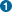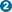## Name

THERMO — Set the temperature range for calculating thermodynamic properties.

## Synopsis

` THERMO(`nnn`) `
` THERMO(`nnn,mmm`) `
` THERMO(`nnn,mmm,ooo`) `

## Description

The calculation of thermodynamic properties is now automatic for calculations in which the Hessian matrix is computed, i.e. FORCE, IRC, NEWTON, or LTRD. The computed properties include internal energy, heat capacity, partition function, and entropy to be calculated for translational, rotational, and vibrational degrees of freedom. Special situations such as linear systems and transition states are automatically accommodated. The TRANS keyword may be used to remove modes corresponding to internal rotation that are present in some molecules.

By default values of the temperature range is assumed (200K to 400K in steps of 20 degrees). The THERMO keyword can be used to override these default values. (Caution: The approximations used in calculating the thermodynamic properties are invalid below 100K.)

### THERMO(`nnn`)

The thermodynamic quantities for a 200 degree range of temperatures, starting at nnn Kelvin in steps of 20 degrees, will be calculated.

### THERMO(`nnn,mmm`)

The thermodynamic quantities for the temperature range limited by a lower bound of nnn Kelvin and an upper bound of mmm Kelvin will be calculated. The step size is calculated to give approximately 20 points, and a reasonable value for the step. The size of the step will be 1, 2, or 5, times a power of 10.

### THERMO(`nnn,mmm,ooo`)

The thermodynamic quantities for the temperature range limited by a lower bound of nnn Kelvin and an upper bound of mmm Kelvin will be calculated. The step size is given by ooo Kelvin and cannot be less than 1K.

none

### Requires:

FORCE, IRC, NEWTON, or LTRD

none

ROT and TRANS

## Examples:

### Input File (`properties/prop_thermo_trans.dat`) :

```  am1 rhf singlet t=auto truste force rot=2 trans=2 print=1p-xylene
Thermo with TRANS=2 to remove internal rotation of methyls
C              0.000000  0    0.000000  0    0.000000  0    0    0    0C              1.395052  1    0.000000  0    0.000000  0    1    0    0
C              1.396919  1  120.474038  1    0.000000  0    2    1    0
C              1.396919  1  119.051836  1   -0.106881  1    3    2    1
C              1.395052  1  120.474038  1    0.106881  1    4    3    2
C              1.396919  1  120.474038  1    0.108409  1    1    2    3
H              1.100167  1  119.542900  1  179.980911  1    1    6    5
H              1.100167  1  119.983003  1 -179.979770  1    2    1    6
H              1.100167  1  119.542900  1 -179.980911  1    4    3    2
H              1.100167  1  119.983003  1  179.979770  1    5    4    3
C              1.480664  1  120.472114  1 -179.388508  1    3    2    1
H              1.119254  1  109.937276  1   89.635686  1   11    3    2
H              1.116344  1  111.043245  1 -149.505323  1   11    3    2
H              1.116344  1  111.043245  1  -31.223306  1   11    3    2
C              1.480664  1  120.472114  1 -179.388508  1    6    1    2
H              1.116344  1  111.043245  1 -149.505323  1   15    6    1
H              1.116344  1  111.043245  1  -31.223306  1   15    6    1
H              1.119254  1  109.937276  1   89.635686  1   15    6    1
0              0.000000  0    0.000000  0    0.000000  0    0    0    0
```
 The keyword FORCE calls for a frequency calculation (in Cartesians) along with the generation of thermodynamic data. In order to obtain correct results for the thermodynamic energy partition, the symmetry number for the point group must be given. The TS’s point group is C2h, requiring ROT=2. The keyword TRANS=2 is optional as the new AMPAC automatically accounts for the presence of two negative eigenvalues This geometry was pre-optimized. If the geometry is not pre-optimized, then one of AMPAC’s geometry optimizers (energy minimizers: TRUSTE, EF, NEWTON, BFGS, DFP; gradient minimizers: TRUSTG, TS, or LTRD) should be specified along with FORCE, so that the geometry will be optimized just prior to computing frequencies.